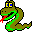#Boost C++ Libraries

...one of the most highly regarded and expertly designed C++ library projects in the world.

##Function template circle_graph_layout

boost::circle_graph_layout — Layout the graph with the vertices at the points of a regular n-polygon.

## Synopsis

```template<typename VertexListGraph, typename PositionMap, typename Radius>
void circle_graph_layout(const VertexListGraph & g, PositionMap position,

## Where Defined

boost/graph/circle_layout.hpp

## Description

The distance from the center of the polygon to each point is determined by the radius parameter. The position parameter must be an Lvalue Property Map whose value type is a class type containing x and y members that will be set to the x and y coordinates.

## Parameters

IN: const VertexListGraph& g
The graph object on which the algorithm will be applied. The type VertexListGraph must be a model of Vertex List Graph.
Python: The parameter is named graph.
OUT: PositionMap position
This property map is used to store the position of each vertex. The type PositionMap must be a model of Writable Property Map, with the graph's edge descriptor type as its key type. The value type of this property map should be assignable from the type Radius.
Python: The position map must be a vertex_point2d_map for the graph.
Python default: graph.get_vertex_point2d_map("position")